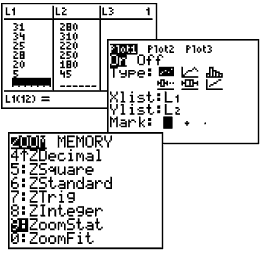Scatter Plots

A scatter plot is a graph used to determine whether
there is a relationship between paired data.

 In many real-life situations, scatter plots follow patterns that are approximately linear.  If y tends to increase as x increases, then the paired data are said to be a positive correlation.  If y tends to decrease as x increases, the paired data are said to be a negative correlation.  If the points show no linear pattern, the paired data are said to have relatively no correlation.To set up a scatter plot:
Clear (or deactivate) any entries in "Y=" before you begin.

 1.  Enter the X data values in L1.  Enter the Y data values in L2, being careful that each X data value and its matching Y data value are entered on the same horizontal line.   (See Basic Commands for entering data.)2.  Activate the scatter plot.  Press 2nd STATPLOT and choose #1 PLOT 1.   You will see the screen at the right.  Be sure the plot is ON, the scatter plot icon is highlighted, and that the list of the X data values are next to Xlist, and the list of the Y data values are next to Ylist.  Choose any of the three marks. 3.  To see the scatter plot, press ZOOM and #9 ZoomStat.  Hitting TRACE and right arrow will move along the data points. 4.  To turn the scatter plot off, when you are finished with this problem:        Method 1:   Go to the Y= screen.  Arrow up onto the PLOT highlighted at the top of the screen.                            Press ENTER to turn it off.        Method 2:   Go to STAT PLOT (above Y=).  Choose your PLOT location.  Arrow to OFF.                            Press ENTER to turn it off.   Follow-up: *  At this point, the graph may be observed for the existence of a positive, negative or no correlation between the data. *  A line of best fit can be calculated manually.     1. Select two points that you feel would give a line that fits the data.     2. Using your knowledge of equations of lines and slope, write the equation of your line.     3. Enter this equation into Y1 and graph.     4. How well does the line fit the data?     5. Use your line to make predictions. *  Or a line of best fit can be calculated "using the calculator".     See Line of Best Fit.Finding Your Way Around TABLE of  CONTENTS